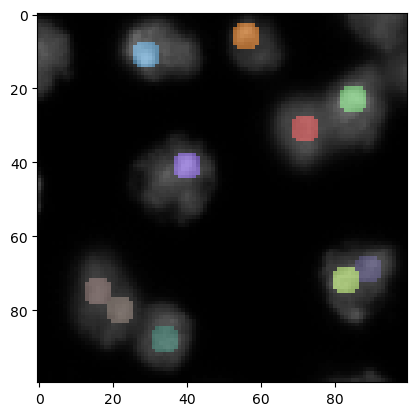# Local maxima detection#

For detecting local maxima, pixels surrounded by pixels with lower intensity, we can use some functions in scikit-image and clesperanto.

from skimage.feature import peak_local_max
import pyclesperanto_prototype as cle
from skimage.filters import gaussian
import matplotlib.pyplot as plt


We start by loading an image and cropping a region for demonstration purposes. We used image set BBBC007v1 image set version 1 (Jones et al., Proc. ICCV Workshop on Computer Vision for Biomedical Image Applications, 2005), available from the Broad Bioimage Benchmark Collection [Ljosa et al., Nature Methods, 2012].

image = imread("../../data/BBBC007_batch/A9 p7d.tif")[-100:, 0:100]

cle.imshow(image)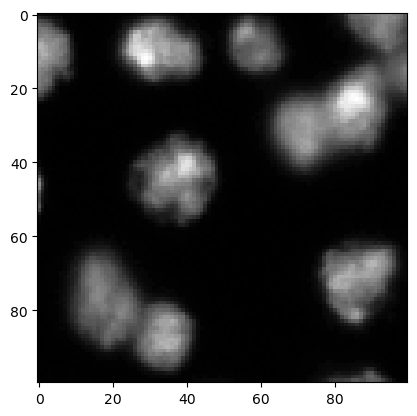## Preprocessing#

A common preprocessing step before detecting maxima is blurring the image. This makes sense to avoid detecting maxima that are just intensity variations resulting from noise.

preprocessed = gaussian(image, sigma=2, preserve_range=True)

cle.imshow(preprocessed)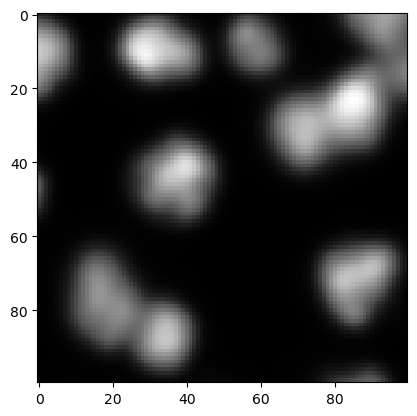## peak_local_max#

The peak_local_max function allows to detect maxima which have higher intensity than surrounding pixels and other maxima according to a define threshold.

coordinates = peak_local_max(preprocessed, threshold_abs=5)
coordinates

array([[23, 85],
[11, 29],
[41, 40],
[88, 34],
[72, 83],
[69, 89],
[31, 72],
[75, 16],
[80, 22],
[ 6, 56]], dtype=int64)


These coordinates can be visualized using matplotlib’s plot function.

cle.imshow(preprocessed, continue_drawing=True)
plt.plot(coordinates[:, 1], coordinates[:, 0], 'r.')

[<matplotlib.lines.Line2D at 0x2309908fbb0>]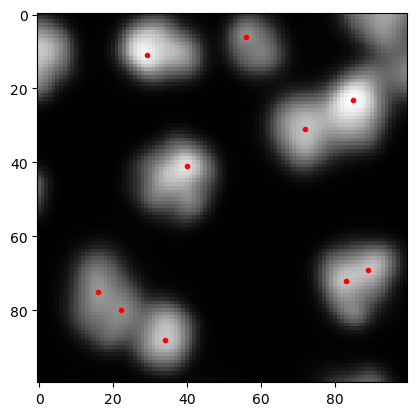If there are too many maxima detected, one can modify the results by changing the sigma parameter of the Gaussian blur above or by changing the threshold passed to the peak_local_max function.

## detect_maxima_box#

The function peak_local_max tends to take long time, e.g. when processing large 3D image data. Thus, an alternaive shall be introduced: clesperanto’s detect_maxima_box is an image filter that sets pixels to value 1 in case surrounding pixels have lower intensity. It typically performs fast also on large 3D image data.

local_maxima_image = cle.detect_maxima_box(preprocessed)
local_maxima_image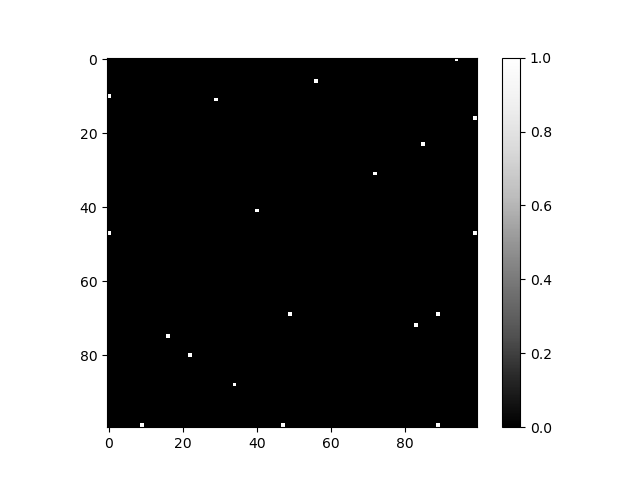cle._ image
 shape (100, 100) dtype uint8 size 9.8 kB min 0.0 max 1.0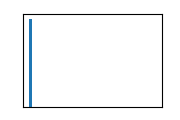Obviously, it results in a binary image. This binary image can be converted to a label image by labeling individual spots with different numbers. From this label image, we can remove maxima detected at image borders, which might be useful in this case.

all_labeled_spots = cle.label_spots(local_maxima_image)

labeled_spots = cle.exclude_labels_on_edges(all_labeled_spots)
labeled_spots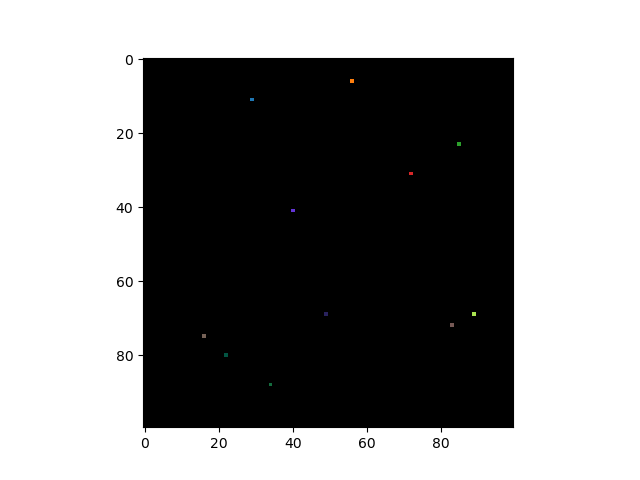cle._ image
 shape (100, 100) dtype uint32 size 39.1 kB min 0.0 max 11.0

To visualize these spots on the original image, it might make sense to increase the size of the spots - just for visualization purposes.

label_visualization = cle.dilate_labels(labeled_spots, radius=3)

cle.imshow(preprocessed, continue_drawing=True)
cle.imshow(label_visualization, labels=True, alpha=0.5)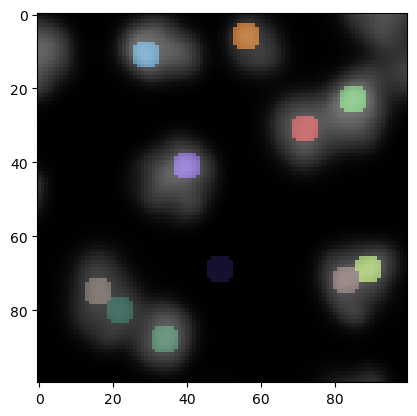In the lower center of this image we see now a local maximum that has been detected in the background. We can remove those maxima in lower intensity regions by thresholding.

binary_image = cle.threshold_otsu(preprocessed)
binary_image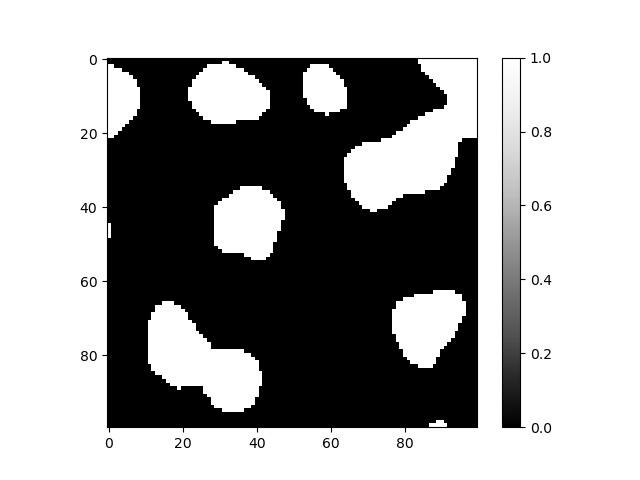cle._ image
 shape (100, 100) dtype uint8 size 9.8 kB min 0.0 max 1.0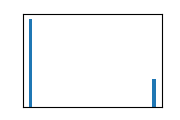We can now exclude labels from the spots image where the intensity in the binary image is not within range [0..1].

final_spots = cle.exclude_labels_with_map_values_out_of_range(
binary_image,
labeled_spots,
minimum_value_range=1,
maximum_value_range=1
)
final_spots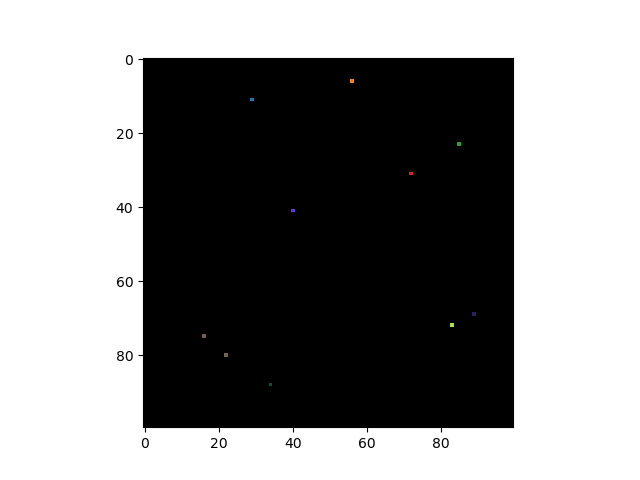cle._ image
 shape (100, 100) dtype uint32 size 39.1 kB min 0.0 max 10.0

We can then visualize the spots again using the strategy introduced above, but this time on the original image.

label_visualization2 = cle.dilate_labels(final_spots, radius=3)

cle.imshow(image, continue_drawing=True)
cle.imshow(label_visualization2, labels=True, alpha=0.5)